# Interpreting ACF and PACF Plots for Time Series Forecasting | by Leonie Monigatti | Aug, 2022

## How to determine the order of AR and MA modelsImage by the author via Kaggle

Autocorrelation analysis is an important step in the Exploratory Data Analysis of time series forecasting. The autocorrelation analysis helps detect patterns and check for randomness. It’s especially important when you intend to use an autoregressive–moving-average (ARMA) model for forecasting because it helps to determine its parameters. The analysis involves looking at the Autocorrelation Function (ACF) and Partial Autocorrelation Function (PACF) plots.

This article helps you build an intuition for interpreting ACF and PACF plots.

This article helps you build an intuition for interpreting these ACF and PACF plots. We’ll briefly go over the fundamentals of the ACF and PACF. However, as the focus lies in the interpretation of the plots, a detailed discussion of the underlying mathematics is beyond the scope of this article. We’ll refer to other resources instead.

This article is a revisited version of my Kaggle Notebook, which was originally published in December 2021. You can download or fork the code there.

The ACF and PACF plots are used to figure out the order of AR, MA, and ARMA models. In this section, we’ll only briefly touch on the relevant terms. For detailed explanations, we’ll refer to other resources.

## Auto-Regressive and Moving Average Models

Auto-Regressive Model

The Auto-Regressive (AR) model assumes that the current value (y_t) is dependent on previous values (y_(t-1), y_(t-2), …). Because of this assumption, we can build a linear regression model.

To figure out the order of an AR model, you need to look at the PACF.

Moving Average Model

The Moving Average (MA) model assumes that the current value (y_t) is dependent on the error terms including the current error (𝜖_t, 𝜖_(t-1),…). Because error terms are random, there’s no linear relationship between the current value and the error terms.

To figure out the order of an MA model, you need to look at the ACF.

## Precondition: Stationarity

ACF and PACF assume stationarity of the underlying time series.

## Autocorrelation Function (ACF) and Partial Autocorrelation Function (PACF)

The ACF and PACF are used to figure out the order of AR, MA, and ARMA models.

If you need some introduction to or a refresher on the ACF and PACF, I recommend the following video: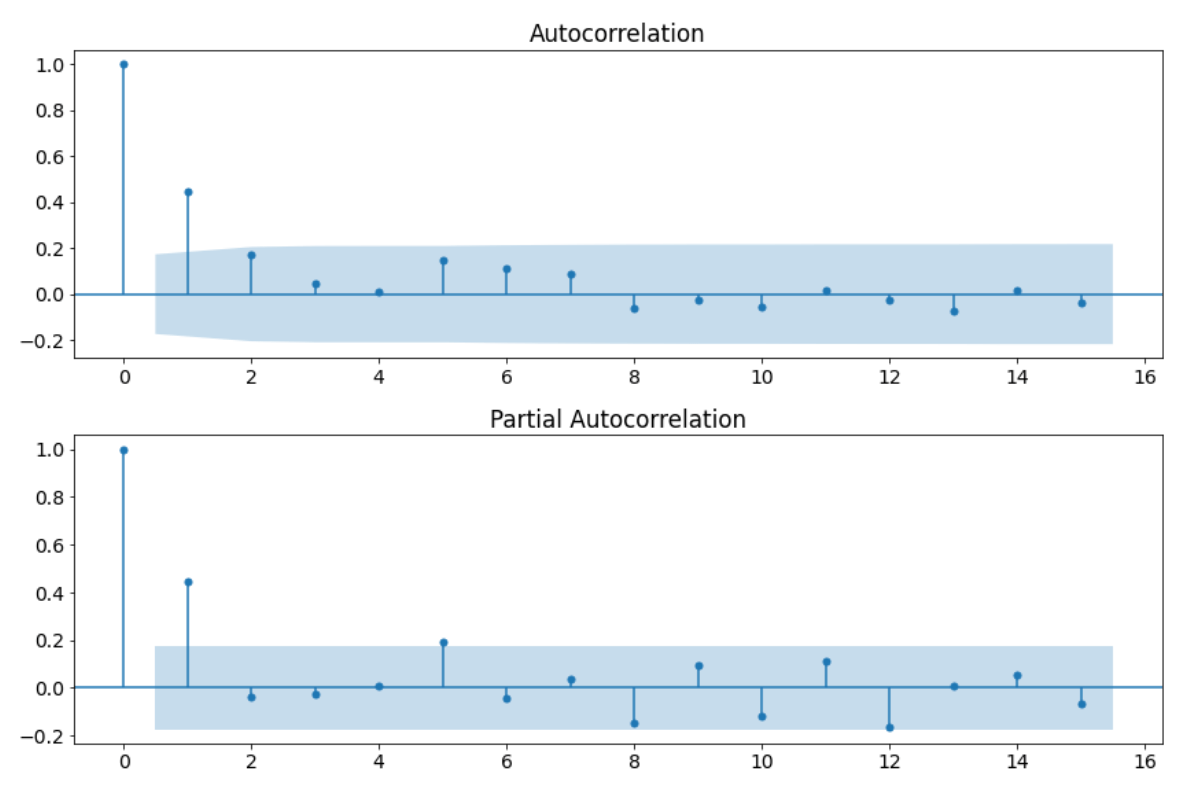Example of an ACF and a PACF plot. (Image by the author via Kaggle)

In this section, we’ll look at a few time series examples and look at:

• What the ACF and PACF plots look like
• How to determine whether to model the time series with an AR or MA model
• How to determine the order of the AR or MA model
• How to find the parameters of the AR or MA model

## AR(1) Process

The following time series is an AR(1) process with 128 timesteps and `alpha_1 = 0.5`. It meets the precondition of stationarity.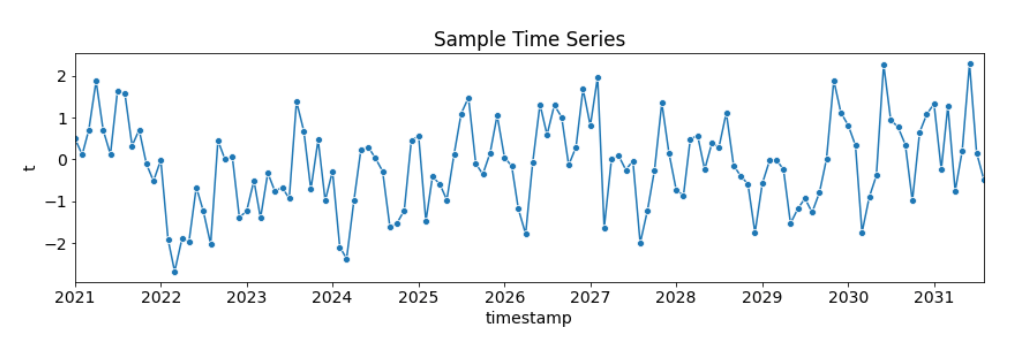Fictional Sample Time Series: AR(1) Process with alpha_1 = 0.5 (Image by the author via Kaggle)

The following figure shows the resulting ACF and PACF plots:ACF and a PACF plot of the AR(1) process. (Image by the author via Kaggle)

We can make the following observations:

• There are several autocorrelations that are significantly non-zero. Therefore, the time series is non-random.
• High degree of autocorrelation between adjacent (lag = 1) in PACF plot
• Geometric decay in ACF plot

Based on the above table, we can use an AR(1) model to model this process.

With AR(p=1), the formula

can be rewritten to the following:

To find the parameter `alpha_1` we fit the AR model as follows:

`from statsmodels.tsa.ar_model import AutoRegar_model = AutoReg(X_train, lags = 1).fit()ar_model.summary()`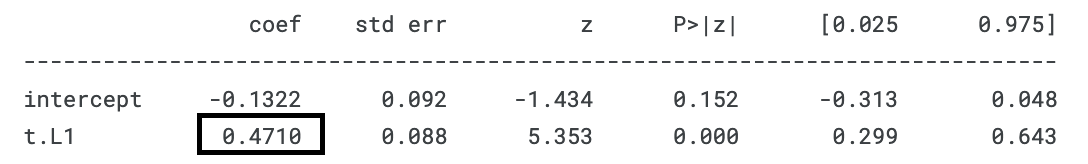Parameter fitted by the AR model. (Image by the author via Kaggle)

As you can see, the AR(1) model fits an `alpha_1 = 0.4710`, which is quite close to the `alpha_1 = 0.5` that we have set.

## AR(2) Process

The following time series is an AR(2) process with 128 timesteps, `alpha_1 = 0.5`, and `alpha_2 = -0.5`. It meets the precondition of stationarity.Fictional Sample Time Series: AR(2) Process with alpha_1 = 0.5 and alpha_2 = -0.5 (Image by the author via Kaggle)

The following figure shows the resulting ACF and PACF plots:ACF and a PACF plot of the AR(2) process. (Image by the author via Kaggle)

We can make the following observations:

• There are several autocorrelations that are significantly non-zero. Therefore, the time series is non-random.
• High degree of autocorrelation between adjacent (lag = 1) and near-adjacent (lag = 2) observations in PACF plot
• Geometric decay in ACF plotImage by the author via Kaggle inspired by 

Based on the above table, we can use an AR(2) model to model this process.

With AR(p=2), the formula

can be rewritten to the following:

To find the parameters `alpha_1` and `alpha_2` we fit the AR model as follows:

`from statsmodels.tsa.ar_model import AutoRegar_model = AutoReg(X_train, lags = 2).fit()ar_model.summary()`Parameters fitted by the AR model. (Image by the author via Kaggle)

As you can see, the AR(2) model fits an `alpha_1 = 0.5191` and `alpha_2 = -0.5855`, which is quite close to the `alpha_1 = 0.5` and `alpha_2 = -0.5` that we have set.

## MA(1) Process

The following time series is an MA(1) process with 128 timesteps and `beta_1 = 0.5`. It meets the precondition of stationarity.Fictional Sample Time Series: MA(1) Process with beta_1 = 0.5 (Image by the author via Kaggle)

The following figure shows the resulting ACF and PACF plots: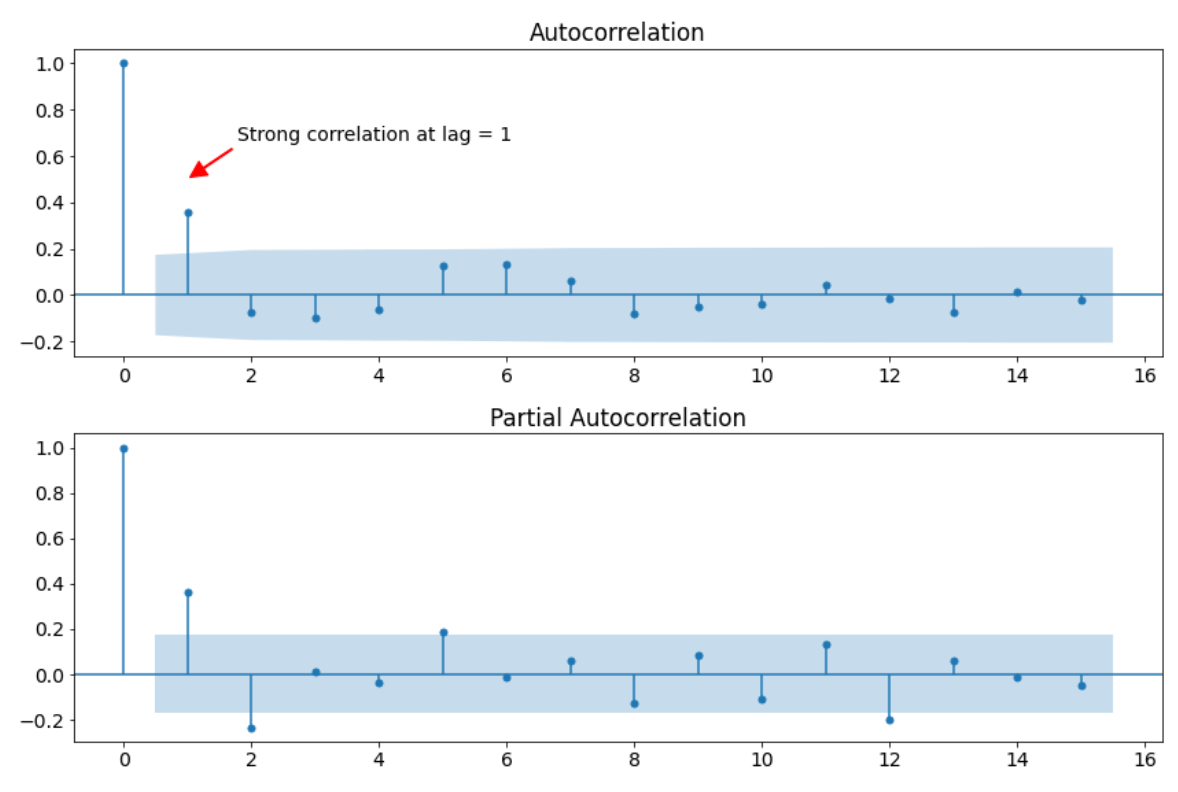ACF and a PACF plot of the MA(1) process. (Image by the author via Kaggle)

We can make the following observations:

• There are several autocorrelations that are significantly non-zero. Therefore, the time series is non-random.
• High degree of autocorrelation between adjacent (lag = 1) in ACF plot
• Geometric decay in PACF plotImage by the author via Kaggle inspired by 

Based on the above table, we can use an MA(1) model to model this process.

With MA(q=1), the formula

can be rewritten to the following:

To find the parameter `beta_1` we fit the MA model as follows:

`from statsmodels.tsa.arima_model import ARMAma_model = ARMA(X_train, order = (0, 1)).fit()ma_model.summary()`Parameter fitted by the (AR)MA model. (Image by the author via Kaggle)

As you can see, the MA(1) model fits a `beta_1 = 0.5172`, which is quite close to the `beta_1 = 0.5` that we have set.

## MA(2) Process

The following time series is an MA(2) process with 128 timesteps and `beta_1 = 0.5` and `beta_2 = 0.5`. It meets the precondition of stationarity.Fictional Sample Time Series: MA(2) Process with beta_1 = 0.5 und beta_2 = 0.5 (Image by the author via Kaggle)

The following figure shows the resulting ACF and PACF plots:ACF and a PACF plot of the MA(2) process. (Image by the author via Kaggle)

We can make the following observations:

• There are several autocorrelations that are significantly non-zero. Therefore, the time series is non-random.
• High degree of autocorrelation between adjacent (lag = 1) and near-adjacent (lag = 2) observations in ACF plot
• Geometric decay in PACF plotImage by the author via Kaggle inspired by 

Based on the above table, we can use an MA(2) model to model this process.

With MA(q=2), the formula

can be rewritten to the following:

To find the parameters `beta_1` and `beta_2` we fit the MA model as follows:

`from statsmodels.tsa.arima_model import ARMAma_model = ARMA(X_train, order = (0, 2)).fit()ma_model.summary()`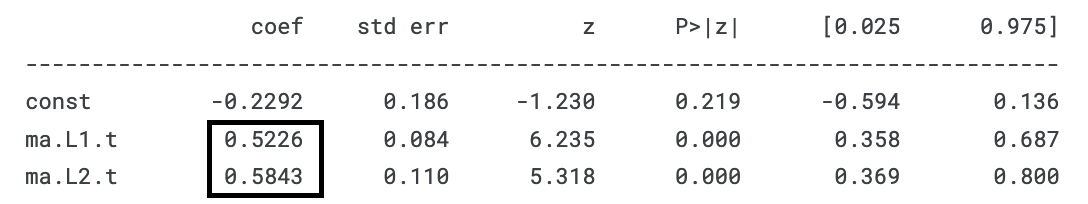Parameters fitted by the (AR)MA model. (Image by the author via Kaggle)

As you can see, the MA(2) model fits a `beta_1 = 0.5226` and `beta_2 = 0.5843`, which is quite close to the `beta_1 = 0.5` and `beta_2 = 0.5` that we have set.

## Periodical

The following time series is periodical with T=12. It consists of 48 timesteps.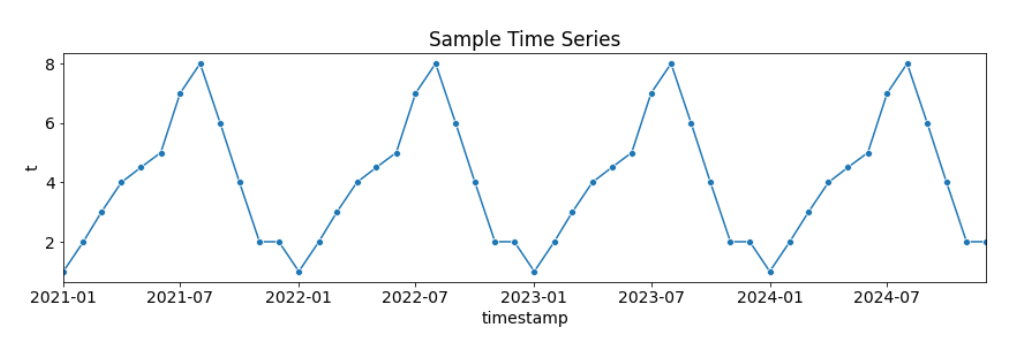Fictional Sample Time Series: Periodical with T=12 (Image by the author via Kaggle)

The following figure shows the resulting ACF and PACF plots: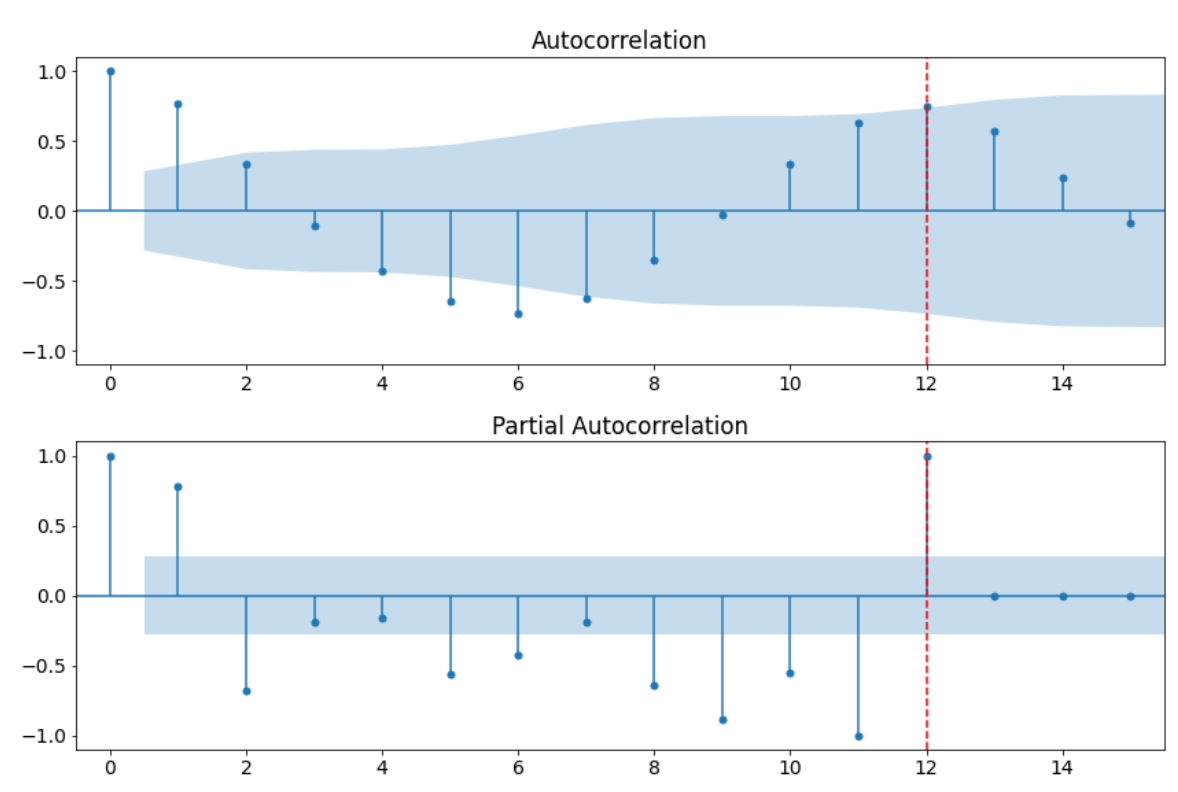ACF and a PACF plot of the periodical process. (Image by the author via Kaggle)

We can make the following observations:

• There are several autocorrelations that are significantly non-zero. Therefore, the time series is non-random.
• High degree of autocorrelation between adjacent (lag = 1) and near-adjacent observations in PACF plot
• From both the ACF and PACF plot, we can see a strong correlation with the adjacent observation (lag = 1) and also at a lag of 12, which is the value of T.Image by the author via Kaggle inspired by 

With AR(p=12), the formula

can be rewritten to the following:

To find the parameters `alpha_1` through `alpha_12` we fit the AR model as follows:

`from statsmodels.tsa.ar_model import AutoRegar_model = AutoReg(X_train, lags = 12).fit()ar_model.summary()`Parameters fitted by the AR model. (Image by the author via Kaggle)

As you can see, the MA(2) model fits the parameters `alpha_1..11 = -0.0004` and `alpha_12 = 0.9996`, which is quite close to the `alpha_1..11 = 0` and `alpha_12 = 1` that we have set.

With these parameters, the formula can be rewritten as shown below:

## White Noise

The following time series is random. It consists of 48 timesteps.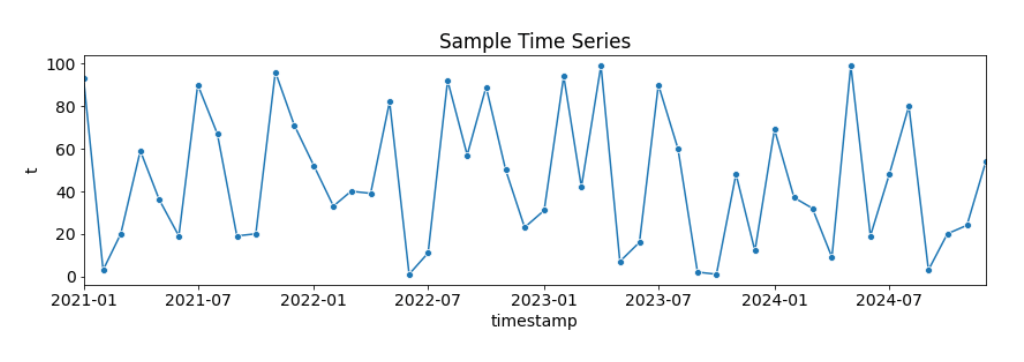Fictional Sample Time Series: White Noise (Image by the author via Kaggle)

The following figure shows the resulting ACF and PACF plots: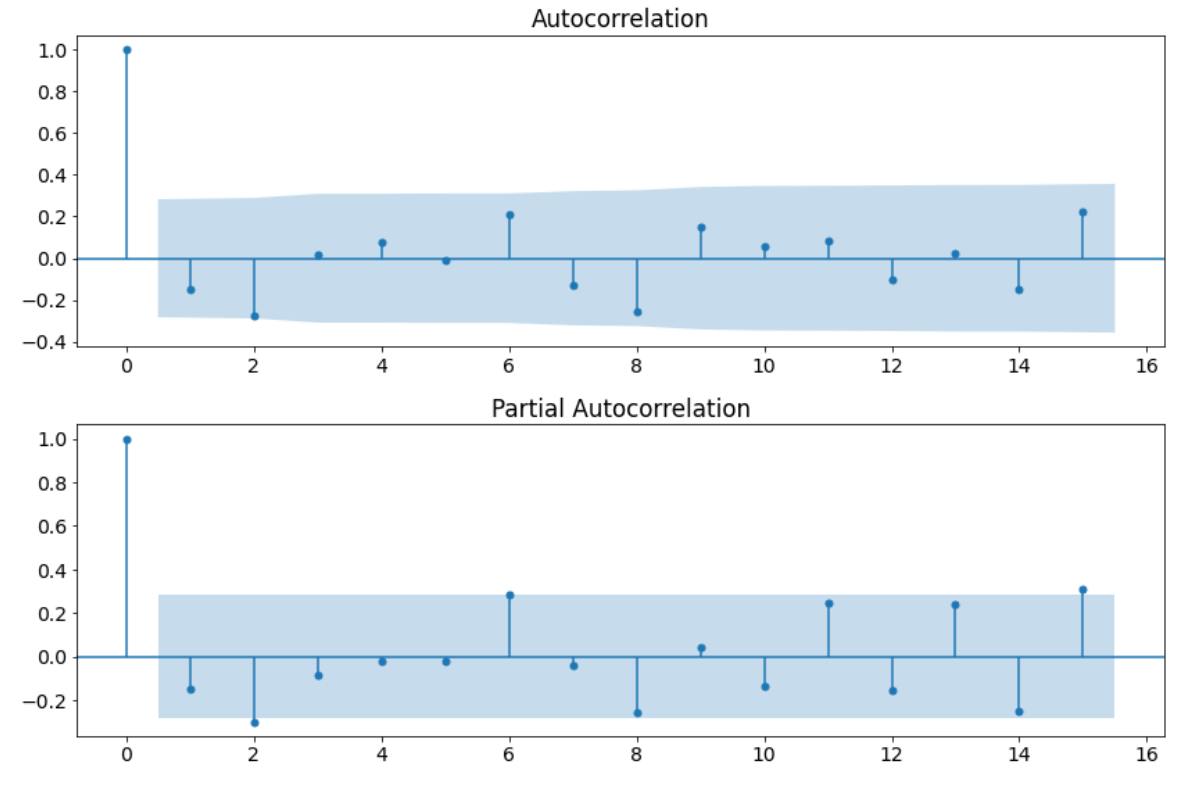ACF and a PACF plot of the white noise. (Image by the author via Kaggle)

We can make the following observation:

• There’s only one autocorrelation that is significantly non-zero at a lag of 0. Therefore, the time series is random.

Modeling white noise is difficult because we can’t retrieve any parameters from the ACF and PACF plots.

In this article, we looked at various examples of AR and MA processes, periodical time series, and white noise to help you build an intuition for interpreting ACF and PACF plots.